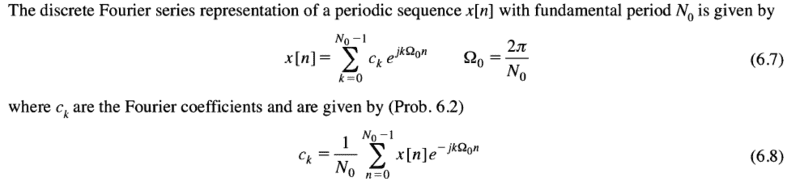# Solving for the Real and Imaginary Number

## Homework Statement

Find the 4th Coefficient in a sample of 4 discrete time Fourier Series coefficients in a real time valued periodic sequence. k = 0,1,2,3

a_k = {3, 1-2j, -1, ?}

## Homework Equations[/B]

## The Attempt at a Solution

Step 1: (1-2j)e^(j*.5pi*n) +a_3 e ^ -(j*.5pi*n) + 3 + (-1)^(n+1)

Step 2: [(1-2j)(cos (pi/2)n + j sin (pi/2)n) + a_3 (cos (pi/2)n)-j sin (pi/2)n]

Solving for a_3 in the final step is where I am confused.

#### Attachments

•